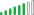cancel
Showing results for
Did you mean:Helper II

## Total Column Sum of measure

Hello All,

Does anyone know how to change the below formula to get the total column to total properly? That is to sum Nov + Dec?
I finally got the column to total (Nov and Dec) however now the total column does not total.

Here is the formula I used:

Forecast =
VAR ForecastTable=
SUMMARIZE(
Data,
Data[Partner & Payment Type]
),
"Rate", [Forecast - Rate],
"FFS", [Forecast - FFS Sum]
)
RETURN
SUMX(ForecastTable,
([Rate] * [Population]) + [FFS]
)1 ACCEPTED SOLUTIONSuper User

Hi @Kryssy

``````Forecast =
VAR ForecastTable =
SUMMARIZE ( Data, Data[Partner & Payment Type], 'Date'[Month] ),
"Rate", [Forecast - Rate],
"FFS", [Forecast - FFS Sum]
)
RETURN
SUMX ( ForecastTable, ( [Rate] * [Population] ) + [FFS] )``````
3 REPLIES 3Super User

Hi @Kryssy

``````Forecast =
VAR ForecastTable =
SUMMARIZE ( Data, Data[Partner & Payment Type], 'Date'[Month] ),
"Rate", [Forecast - Rate],
"FFS", [Forecast - FFS Sum]
)
RETURN
SUMX ( ForecastTable, ( [Rate] * [Population] ) + [FFS] )``````Helper II

Thanks Again!!!!!!Solution Supplier

Hi @Kryssy ,

Can you share sample pbix after removing sensitive data, so that we can check the Total, Nov and Dec column structure in your report?

Thanks!

Inogic Professional Service Division

Power Platform/Dynamics 365 CRM

Drop an email at crm@inogic.com

Service:  http://www.inogic.com/services/

Power Platform/Dynamics 365 CRM Tips and Tricks:  http://www.inogic.com/blog/